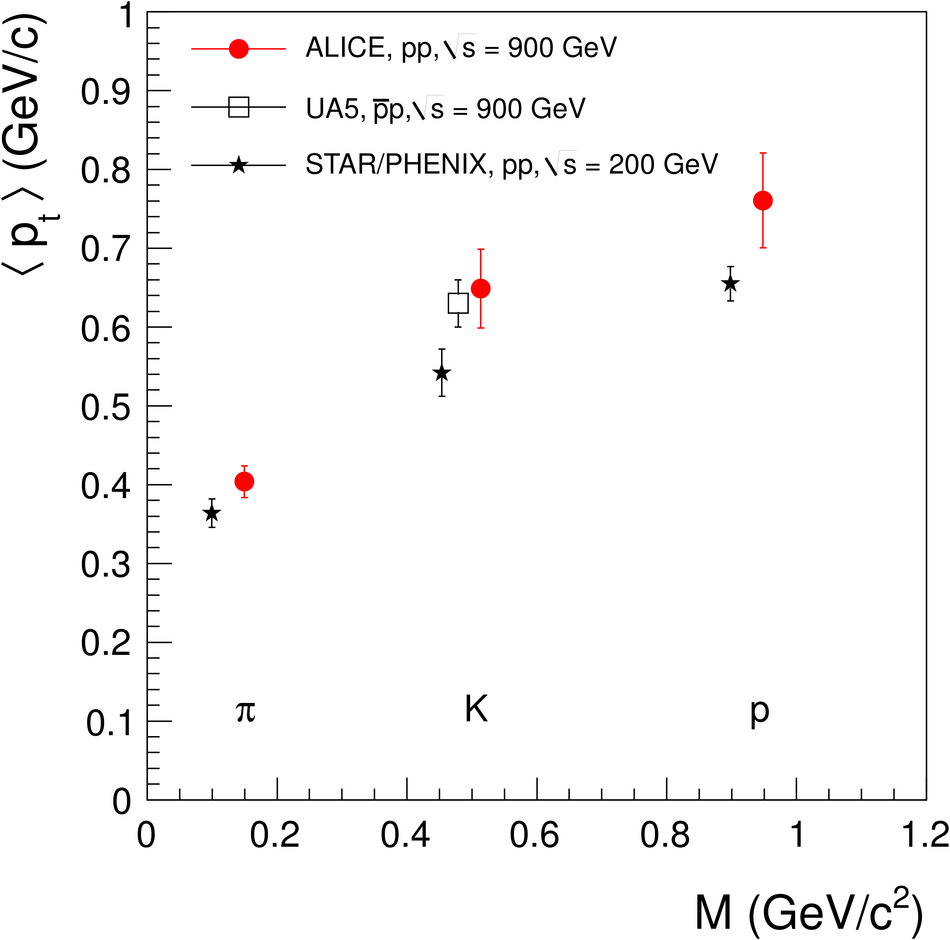# Figure 15

 Mean $\pt$ as a function of the mass of the emitted particle in pp collisions at 900 GeV (ALICE, red solid circles, statistical and systematic errors) compared to results at $\sqrt{s} = 200~$GeV (star markers, average values of the results from the STAR and the PHENIX Collaborations [32,33]) and $\rm\overline{p}$p reactions at $\sqrt{s} = 900~$GeV (open squares). Some data points are displaced for clarity.